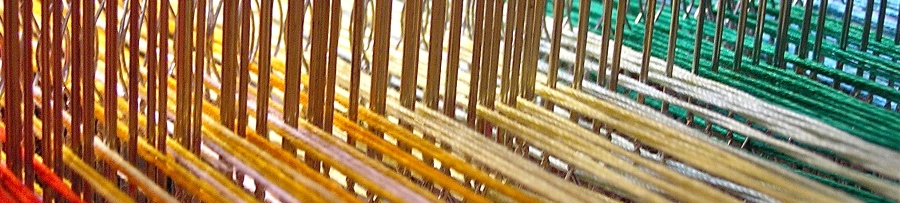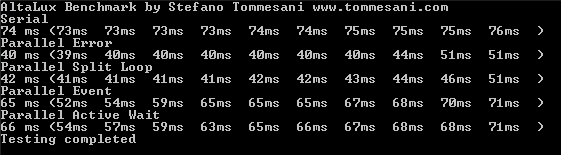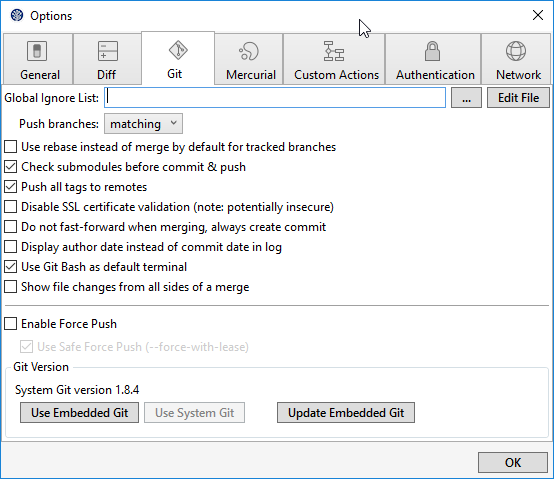# Stefano Tommesani

•••## Parallel loops in the AltaLux kernelThe AltaLux plugin for IrfanView is now open-source (here is the GitHub link) and it is worth analyzing the different methods used for parallelizing the computational kernel. The filter factory, contained in the CAltaLuxFilterFactory files, can create one of four possible instance types (actually there's a fifth one that you will never want to use, as we will see later). Most of the plumbing code is hosted in a base abstract class, named CBaseAltaLuxFilter, and there are four specific classes that implement the virtual Run method in different ways:

1. CSerialAltaLuxFilter
2. CParallelSplitLoopAltaLuxFilter
3. CParallelEventAltaLuxFilter
4. CParallelActiveWaitAltaLuxFilter

Let's start with the first one, the serial implementation, to have an idea of how the code works. Here is the whole Run method:

```/// <summary>
/// processes incoming image
/// </summary>
/// <returns>error code, refer to AL_XXX codes</returns>
/// <remarks>
/// serial code, used as reference for parallel implementations
/// [Filter processing of full resolution image] in [1.245 seconds]
/// </remarks>
int CSerialAltaLuxFilter::Run()
{
if (ClipLimit == 1.0)
return AL_OK;      //< is OK, immediately returns original image

PixelType *pImage = (PixelType *)ImageBuffer;

/// pulMapArray is pointer to mappings
unsigned int *pulMapArray = new unsigned int[NumHorRegions * NumVertRegions * NUM_GRAY_LEVELS];
if (pulMapArray == 0)
return AL_OUT_OF_MEMORY;      //< not enough memory

/// region pixel count
unsigned int NumPixels = (unsigned int)RegionWidth * (unsigned int)RegionHeight;    //< region pixel count

unsigned int ulClipLimit;        //< clip limit
if (ClipLimit > 0.0)
{
/// calculate actual cliplimit
ulClipLimit = (unsigned int) (ClipLimit * (RegionWidth * RegionHeight) / NUM_GRAY_LEVELS);
ulClipLimit = (ulClipLimit < 1UL) ? 1UL : ulClipLimit;
}
else
ulClipLimit = 1UL<<14;          //< large value, do not clip (AHE)

/// Interpolate greylevel mappings to get CLAHE image
for (int uiY = 0; uiY <= NumVertRegions; uiY++)
{
// first half
PixelType* pImPointer = pImage;
if (uiY > 0)
pImPointer += ((RegionHeight >> 1) + ((uiY - 1) * RegionHeight)) * OriginalImageWidth;

if (uiY < NumVertRegions)
{
/// calculate greylevel mappings for each contextual region
for (unsigned int uiX = 0; uiX < NumHorRegions; uiX++, pImPointer += RegionWidth)
{
unsigned int *pHistogram = &pulMapArray[NUM_GRAY_LEVELS * (uiY * NumHorRegions + uiX)];
MakeHistogram(pImPointer, pHistogram);
ClipHistogram(pHistogram, ulClipLimit);
MapHistogram(pHistogram, NumPixels);
}
}
// second half
unsigned int uiSubX, uiSubY;    //< size of subimages
unsigned int uiXL, uiXR, uiYU, uiYB;    //< auxiliary variables interpolation routine

pImPointer = pImage;
if (uiY > 0)
pImPointer += ((RegionHeight >> 1) + ((uiY - 1) * RegionHeight)) * OriginalImageWidth;

if (uiY == 0)
{
/// special case: top row
uiSubY = RegionHeight >> 1;
uiYU = 0;
uiYB = 0;
} else {
if (uiY == NumVertRegions)
{
/// special case: bottom row
uiSubY = (RegionHeight >> 1) + (OriginalImageHeight - ImageHeight);
uiYU = NumVertRegions - 1;
uiYB = uiYU;
} else {
/// default values
uiSubY = RegionHeight;
uiYU = uiY - 1;
uiYB = uiY;
}
}

for (unsigned int uiX = 0; uiX <= NumHorRegions; uiX++)
{
if (uiX == 0)
{
/// special case: left column
uiSubX = RegionWidth >> 1;
uiXL = 0;
uiXR = 0;
} else {
if (uiX == NumHorRegions)
{
/// special case: right column
uiSubX = (RegionWidth >> 1) + (OriginalImageWidth - ImageWidth);
uiXL = NumHorRegions - 1;
uiXR = uiXL;
} else {
/// default values
uiSubX = RegionWidth;
uiXL = uiX - 1;
uiXR = uiX;
}
}
unsigned int* pulLU = &pulMapArray[NUM_GRAY_LEVELS * (uiYU * NumHorRegions + uiXL)];
unsigned int* pulRU = &pulMapArray[NUM_GRAY_LEVELS * (uiYU * NumHorRegions + uiXR)];
unsigned int* pulLB = &pulMapArray[NUM_GRAY_LEVELS * (uiYB * NumHorRegions + uiXL)];
unsigned int* pulRB = &pulMapArray[NUM_GRAY_LEVELS * (uiYB * NumHorRegions + uiXR)];

Interpolate(pImPointer, pulLU, pulRU, pulLB, pulRB, uiSubX, uiSubY);

pImPointer += uiSubX;    //< set pointer on next matrix
}
}
delete[] pulMapArray;
return AL_OK;            //< return status OK
}```

## First attempt at parallel processing

The computational kernel can be roughly divided in two parts, that I have marked in the code with the "first half" and "second half" comments. If only each iteration of this loop had no dependencies on previous iterations, writing a parallel version of the loop would be really easy, as a concurrency::parallel_for for the outer loop would gain most of the benefits with little effort... and this is exactly what the fifth implementation (CParallelErrorAltaLuxFilter), the one you will never want to use, does, running twice as fast as the serial version but also producing wrong results:

```concurrency::parallel_for((int)0, (int)(NumVertRegions + 1), [&](int uiY)
```

Still worth having a look, as it represents the optimal speed-up that can be achieved, so that we can measure the performance toll of the synchronization mechanisms used in the other implementations.

Why does the naive parallel version fail? Let's emulate what the algorithm does, when it is processing the block located at column X and row Y, named B(x,y), the second part of the kernel references not only the data produced by the first part of the kernel, but also the results of the first part of the kernel for blocks B(x-1, y-1) and B(x, y-1). So, in the serial implementation, when we are processing block B(x,y), we are 100% sure that blocks on the previous row have already been processed, so we can safely access B(x-1, y-1) and B(x, y-1), but in a multi-thread scenario there is no guarantee that the thread processing the previous row has already completed its job, actually it could well be late, still processing an earlier block of that row. This is why we need to use a synchronization mechanism between loop iterations, so that we access valid data.

## Splitting the loop

The first (working) parallel implementation, named CParallelSplitLoopAltaLuxFilter, divides the kernel in two loops, so that when the second part of the kernel runs, all the computations of the first part are over:

```/// <summary>
/// processes incoming image
/// </summary>
/// <returns>error code, refer to AL_XXX codes</returns>
/// <remarks>
/// parallel code that divides the loop in two and puts a synchronization barrier in the middle
/// to ensure that all data dependencies are resolved before moving to the next steps
/// [Filter processing of full resolution image] in [1.109 seconds]
/// </remarks>
int CParallelSplitLoopAltaLuxFilter::Run()
{
if (ClipLimit == 1.0)
return AL_OK;      //< is OK, immediately returns original image

PixelType *pImage = (PixelType *)ImageBuffer;

/// pulMapArray is pointer to mappings
unsigned int *pulMapArray = new unsigned int[NumHorRegions * NumVertRegions * NUM_GRAY_LEVELS];
if (pulMapArray == 0)
return AL_OUT_OF_MEMORY;      //< not enough memory

/// region pixel count
unsigned int NumPixels = (unsigned int)RegionWidth * (unsigned int)RegionHeight;    //< region pixel count

unsigned int ulClipLimit;        //< clip limit
if (ClipLimit > 0.0)
{
/// calculate actual cliplimit
ulClipLimit = (unsigned int) (ClipLimit * (RegionWidth * RegionHeight) / NUM_GRAY_LEVELS);
ulClipLimit = (ulClipLimit < 1UL) ? 1UL : ulClipLimit;
}
else
ulClipLimit = 1UL<<14;          //< large value, do not clip (AHE)

/// Interpolate greylevel mappings to get CLAHE image
concurrency::parallel_for((int)0, (int)(NumVertRegions + 1), [&](int uiY)
{
PixelType* pImPointer = pImage;
if (uiY > 0)
pImPointer += ((RegionHeight >> 1) + ((uiY - 1) * RegionHeight)) * OriginalImageWidth;

if (uiY < NumVertRegions)
{
/// calculate greylevel mappings for each contextual region
for (unsigned int uiX = 0; uiX < NumHorRegions; uiX++, pImPointer += RegionWidth)
{
unsigned int *pHistogram = &pulMapArray[NUM_GRAY_LEVELS * (uiY * NumHorRegions + uiX)];
MakeHistogram(pImPointer, pHistogram);
ClipHistogram(pHistogram, ulClipLimit);
MapHistogram(pHistogram, NumPixels);
}
}
});

concurrency::parallel_for((int)0, (int)(NumVertRegions + 1), [&](int uiY)
{
unsigned int uiSubX, uiSubY;    //< size of subimages
unsigned int uiXL, uiXR, uiYU, uiYB;    //< auxiliary variables interpolation routine

PixelType* pImPointer = pImage;
if (uiY > 0)
pImPointer += ((RegionHeight >> 1) + ((uiY - 1) * RegionHeight)) * OriginalImageWidth;

if (uiY == 0)
{
/// special case: top row
uiSubY = RegionHeight >> 1;
uiYU = 0;
uiYB = 0;
} else {
if (uiY == NumVertRegions)
{
/// special case: bottom row
uiSubY = (RegionHeight >> 1) + (OriginalImageHeight - ImageHeight);
uiYU = NumVertRegions - 1;
uiYB = uiYU;
} else {
/// default values
uiSubY = RegionHeight;
uiYU = uiY - 1;
uiYB = uiY;
}
}

for (unsigned int uiX = 0; uiX <= NumHorRegions; uiX++)
{
if (uiX == 0)
{
/// special case: left column
uiSubX = RegionWidth >> 1;
uiXL = 0;
uiXR = 0;
} else {
if (uiX == NumHorRegions)
{
/// special case: right column
uiSubX = (RegionWidth >> 1) + (OriginalImageWidth - ImageWidth);
uiXL = NumHorRegions - 1;
uiXR = uiXL;
} else {
/// default values
uiSubX = RegionWidth;
uiXL = uiX - 1;
uiXR = uiX;
}
}
unsigned int* pulLU = &pulMapArray[NUM_GRAY_LEVELS * (uiYU * NumHorRegions + uiXL)];
unsigned int* pulRU = &pulMapArray[NUM_GRAY_LEVELS * (uiYU * NumHorRegions + uiXR)];
unsigned int* pulLB = &pulMapArray[NUM_GRAY_LEVELS * (uiYB * NumHorRegions + uiXL)];
unsigned int* pulRB = &pulMapArray[NUM_GRAY_LEVELS * (uiYB * NumHorRegions + uiXR)];

Interpolate(pImPointer, pulLU, pulRU, pulLB, pulRB, uiSubX, uiSubY);

pImPointer += uiSubX;    //< set pointer on next matrix
}
});

delete[] pulMapArray;
return AL_OK;            //< return status OK
}```

The changes versus the serial version are minimal, we have two parallel loops instead of a single serial one:

```concurrency::parallel_for((int)0, (int)(NumVertRegions + 1), [&](int uiY)
```

The weak spot of this code is that the whole image is processed using the first half of the kernel, and then the same image is processed again with the second half of the kernel, so cache usage is not optimal. Besides, all the loops from the first half must be completed before starting the threads of the second half, so we waste time waiting for the slower thread of the first phase to complete its duty.

## Synchronization with events

Let's try to minimize the shortcomings of the first parallel version with the second one, named CParallelEventAltaLuxFilter:

```/// <summary>
/// processes incoming image
/// </summary>
/// <returns>error code, refer to AL_XXX codes</returns>
/// <remarks>
/// working parallel code with single loop, using Win32 events to protect data dependecies across vertical blocks
/// [Filter processing of full resolution image] in [1.131 seconds]
/// </remarks>
int CParallelEventAltaLuxFilter::Run()
{
if (ClipLimit == 1.0)
return AL_OK;      //< is OK, immediately returns original image

PixelType *pImage = (PixelType *)ImageBuffer;

/// pulMapArray is pointer to mappings
unsigned int *pulMapArray = new unsigned int[NumHorRegions * NumVertRegions * NUM_GRAY_LEVELS];
if (pulMapArray == 0)
return AL_OUT_OF_MEMORY;      //< not enough memory

/// region pixel count
unsigned int NumPixels = (unsigned int)RegionWidth * (unsigned int)RegionHeight;    //< region pixel count

unsigned int ulClipLimit;        //< clip limit
if (ClipLimit > 0.0)
{
/// calculate actual cliplimit
ulClipLimit = (unsigned int) (ClipLimit * (RegionWidth * RegionHeight) / NUM_GRAY_LEVELS);
ulClipLimit = (ulClipLimit < 1UL) ? 1UL : ulClipLimit;
}
else
ulClipLimit = 1UL<<14;          //< large value, do not clip (AHE)

/// Interpolate greylevel mappings to get CLAHE image
// create events for signaling that the first phase is completed
HANDLE FirstPhaseCompleted[MAX_VERT_REGIONS];
for (int i = 0; i < NumVertRegions; i++)
FirstPhaseCompleted[i] = CreateEvent(
NULL,               // default security attributes
TRUE,               // manual-reset event
FALSE,              // initial state is nonsignaled
NULL                // object name
);

concurrency::parallel_for((int)0, (int)(NumVertRegions + 1), [&](int uiY)
{
// first half
PixelType* pImPointer = pImage;
if (uiY > 0)
pImPointer += ((RegionHeight >> 1) + ((uiY - 1) * RegionHeight)) * OriginalImageWidth;

if (uiY < NumVertRegions)
{
/// calculate greylevel mappings for each contextual region
for (unsigned int uiX = 0; uiX < NumHorRegions; uiX++, pImPointer += RegionWidth)
{
unsigned int *pHistogram = &pulMapArray[NUM_GRAY_LEVELS * (uiY * NumHorRegions + uiX)];
MakeHistogram(pImPointer, pHistogram);
ClipHistogram(pHistogram, ulClipLimit);
MapHistogram(pHistogram, NumPixels);
}
}

// signal that the first phase is completed for this horizontal block
if (uiY < NumVertRegions)
SetEvent(FirstPhaseCompleted[uiY]);
// wait for completion of first phase of the previous horizontal block
if (uiY > 0)
DWORD dwWaitResult = WaitForSingleObject(FirstPhaseCompleted[uiY-1], INFINITE);

// second half
unsigned int uiSubX, uiSubY;    //< size of subimages
unsigned int uiXL, uiXR, uiYU, uiYB;    //< auxiliary variables interpolation routine

pImPointer = pImage;
if (uiY > 0)
pImPointer += ((RegionHeight >> 1) + ((uiY - 1) * RegionHeight)) * OriginalImageWidth;

if (uiY == 0)
{
/// special case: top row
uiSubY = RegionHeight >> 1;
uiYU = 0;
uiYB = 0;
} else {
if (uiY == NumVertRegions)
{
/// special case: bottom row
uiSubY = (RegionHeight >> 1) + (OriginalImageHeight - ImageHeight);
uiYU = NumVertRegions - 1;
uiYB = uiYU;
} else {
/// default values
uiSubY = RegionHeight;
uiYU = uiY - 1;
uiYB = uiY;
}
}

for (unsigned int uiX = 0; uiX <= NumHorRegions; uiX++)
{
if (uiX == 0)
{
/// special case: left column
uiSubX = RegionWidth >> 1;
uiXL = 0;
uiXR = 0;
} else {
if (uiX == NumHorRegions)
{
/// special case: right column
uiSubX = (RegionWidth >> 1) + (OriginalImageWidth - ImageWidth);
uiXL = NumHorRegions - 1;
uiXR = uiXL;
} else {
/// default values
uiSubX = RegionWidth;
uiXL = uiX - 1;
uiXR = uiX;
}
}
unsigned int* pulLU = &pulMapArray[NUM_GRAY_LEVELS * (uiYU * NumHorRegions + uiXL)];
unsigned int* pulRU = &pulMapArray[NUM_GRAY_LEVELS * (uiYU * NumHorRegions + uiXR)];
unsigned int* pulLB = &pulMapArray[NUM_GRAY_LEVELS * (uiYB * NumHorRegions + uiXL)];
unsigned int* pulRB = &pulMapArray[NUM_GRAY_LEVELS * (uiYB * NumHorRegions + uiXR)];

Interpolate(pImPointer, pulLU, pulRU, pulLB, pulRB, uiSubX, uiSubY);

pImPointer += uiSubX;    //< set pointer on next matrix
}
});

for (int i = 0; i < NumVertRegions; i++)
CloseHandle(FirstPhaseCompleted[i]);

delete[] pulMapArray;
return AL_OK;            //< return status OK
}```

Now we have a single parallel loop hosting both halves of the kernel, but before moving on accessing blocks on the previous row we check that the the first phase of the previous row was completed:

```// signal that the first phase is completed for this horizontal block
if (uiY < NumVertRegions)
SetEvent(FirstPhaseCompleted[uiY]);
// wait for completion of first phase of the previous horizontal block
if (uiY > 0)
DWORD dwWaitResult = WaitForSingleObject(FirstPhaseCompleted[uiY-1], INFINITE);```

We have to add a bit of overhead at the beginning of the function to prepare the events:

```    // create events for signaling that the first phase is completed
HANDLE FirstPhaseCompleted[MAX_VERT_REGIONS];
for (int i = 0; i < NumVertRegions; i++)
FirstPhaseCompleted[i] = CreateEvent(
NULL,               // default security attributes
TRUE,               // manual-reset event
FALSE,              // initial state is nonsignaled
NULL                // object name
); ```

and at the end to close the handles to the events:

```for (int i = 0; i < NumVertRegions; i++)
CloseHandle(FirstPhaseCompleted[i]);```

So, in the second implementation we don't have to wait for the first phase to be completed on the whole image, ideally the second phase of row 2 can start as soon as the first phase of rows 1 and 2 is over, but we have some overhead waiting for the events. Performance-wise, quite a drop-off, so the overhead seems to offset a better cache access pattern.

## Active waits

Now for the third and final implementation, named CParallelActiveWaitAltaLuxFilter:

```/// <summary>
/// processes incoming image
/// </summary>
/// <returns>error code, refer to AL_XXX codes</returns>
/// <remarks>
/// working parallel code with single loop, using active waits to protect data dependencies
/// [Filter processing of full resolution image] in [1.131 seconds]
/// </remarks>
int CParallelActiveWaitAltaLuxFilter::Run()
{
if (ClipLimit == 1.0)
return AL_OK;      //< is OK, immediately returns original image

PixelType *pImage = (PixelType *)ImageBuffer;

/// pulMapArray is pointer to mappings
unsigned int *pulMapArray = new unsigned int[NumHorRegions * NumVertRegions * NUM_GRAY_LEVELS];
if (pulMapArray == 0)
return AL_OUT_OF_MEMORY;      //< not enough memory

/// region pixel count
unsigned int NumPixels = (unsigned int)RegionWidth * (unsigned int)RegionHeight;    //< region pixel count

unsigned int ulClipLimit;        //< clip limit
if (ClipLimit > 0.0)
{
/// calculate actual cliplimit
ulClipLimit = (unsigned int) (ClipLimit * (RegionWidth * RegionHeight) / NUM_GRAY_LEVELS);
ulClipLimit = (ulClipLimit < 1UL) ? 1UL : ulClipLimit;
}
else
ulClipLimit = 1UL<<14;          //< large value, do not clip (AHE)

/// Interpolate greylevel mappings to get CLAHE image
// create events for signaling that the first phase is completed
__declspec(align(32)) volatile LONG FirstPhaseCompleted[MAX_VERT_REGIONS+1];
for (int i = 0; i <= NumVertRegions; i++)
InterlockedExchange((volatile LONG*)&FirstPhaseCompleted[i], -1);

concurrency::parallel_for((LONG)0, (LONG)(NumVertRegions + 1), [&](LONG uiY)
{
// first half
PixelType* pImPointer = pImage;
if (uiY > 0)
pImPointer += ((RegionHeight >> 1) + ((uiY - 1) * RegionHeight)) * OriginalImageWidth;

if (uiY < NumVertRegions)
{
/// calculate greylevel mappings for each contextual region
for (LONG uiX = 0; uiX < NumHorRegions; uiX++, pImPointer += RegionWidth)
{
unsigned int *pHistogram = &pulMapArray[NUM_GRAY_LEVELS * (uiY * NumHorRegions + uiX)];
MakeHistogram(pImPointer, pHistogram);
ClipHistogram(pHistogram, ulClipLimit);
MapHistogram(pHistogram, NumPixels);
InterlockedExchange((volatile LONG*) &FirstPhaseCompleted[uiY], uiX);
}
}

// second half
unsigned int uiSubX, uiSubY;    //< size of subimages
unsigned int uiXL, uiXR, uiYU, uiYB;    //< auxiliary variables interpolation routine

pImPointer = pImage;
if (uiY > 0)
pImPointer += ((RegionHeight >> 1) + ((uiY - 1) * RegionHeight)) * OriginalImageWidth;

if (uiY == 0)
{
/// special case: top row
uiSubY = RegionHeight >> 1;
uiYU = 0;
uiYB = 0;
} else {
if (uiY == NumVertRegions)
{
/// special case: bottom row
uiSubY = (RegionHeight >> 1) + (OriginalImageHeight - ImageHeight);
uiYU = NumVertRegions - 1;
uiYB = uiYU;
} else {
/// default values
uiSubY = RegionHeight;
uiYU = uiY - 1;
uiYB = uiY;
}
}

for (LONG uiX = 0; uiX <= NumHorRegions; uiX++)
{
if (uiX == 0)
{
/// special case: left column
uiSubX = RegionWidth >> 1;
uiXL = 0;
uiXR = 0;
} else {
if (uiX == NumHorRegions)
{
/// special case: right column
uiSubX = (RegionWidth >> 1) + (OriginalImageWidth - ImageWidth);
uiXL = NumHorRegions - 1;
uiXR = uiXL;
} else {
/// default values
uiSubX = RegionWidth;
uiXL = uiX - 1;
uiXR = uiX;
}
}
if (uiY > 0)
{
while (true)
{
// active waiting until processing of needed blocks on previous line has completed
LONG statePrevLine = InterlockedExchangeAdd((volatile LONG*)&FirstPhaseCompleted[uiY - 1], 0);
if ((statePrevLine > -1)  && ((statePrevLine >= uiXR) || (statePrevLine == (NumHorRegions - 1))))
break;
Sleep(1);
}
}

unsigned int* pulLU = &pulMapArray[NUM_GRAY_LEVELS * (uiYU * NumHorRegions + uiXL)];
unsigned int* pulRU = &pulMapArray[NUM_GRAY_LEVELS * (uiYU * NumHorRegions + uiXR)];
unsigned int* pulLB = &pulMapArray[NUM_GRAY_LEVELS * (uiYB * NumHorRegions + uiXL)];
unsigned int* pulRB = &pulMapArray[NUM_GRAY_LEVELS * (uiYB * NumHorRegions + uiXR)];

Interpolate(pImPointer, pulLU, pulRU, pulLB, pulRB, uiSubX, uiSubY);

pImPointer += uiSubX;    //< set pointer on next matrix
}
});

delete[] pulMapArray;
return AL_OK;            //< return status OK
}```

Now, instead of using events for synchronization, I switch to active waiting over a volatile variable using InterlockedXXX operations. There is a critical bit for initializing the counters:

```__declspec(align(32)) volatile LONG FirstPhaseCompleted[MAX_VERT_REGIONS+1];
for (int i = 0; i <= NumVertRegions; i++)
InterlockedExchange((volatile LONG*)&FirstPhaseCompleted[i], -1);```

So, the loop of the first phase updates the position in the row:

```InterlockedExchange((volatile LONG*) &FirstPhaseCompleted[uiY], uiX);
```

and the loop of the second phase checks the status of the previous row before accessing the data:

```while (true)
{
// active waiting until processing of needed blocks on previous line has completed
LONG statePrevLine = InterlockedExchangeAdd((volatile LONG*)&FirstPhaseCompleted[uiY - 1], 0);
if ((statePrevLine > -1)  && ((statePrevLine >= uiXR) || (statePrevLine == (NumHorRegions - 1))))
break;
Sleep(1);
}```

The best part of this implementation is that I don't have to wait for the previous row to be completely processed by phase 1, just the blocks I need for the second phase of the current block, so if I am processing the first block of row 2, I just need to wait for the first two blocks of the previous row to be ready. On the downside, I am actively waiting for the other computations to complete, so I am definitely burning cycles hoping to get a green light as soon as possible. From the benchmarks, this is the worst performing parallel solution, so there is really no gain from active waits.

## Performance

Summing up, here are three different ways to handle dependencies between loop iterations, each offering different strengths and weaknesses. In the end, the default implementation used by the factory class is the first parallel one, the one that splits the loop in two, as with most frequent image sizes proved to be more performing. Here is the result of running the benchmarking app, processing 4K images, in a 4 cores i5:The parallel split loop is minimally slower than the naive (and wrong) parallelization without synchronization. Adding synchronization by events or active waiting, despite being interesting on paper as potentially more performing, really increase the overhead to a point that the benefit of going parallel instead of serial almost disappears. The project available in GitHub contains an already compiled version of the benchmarking app, so you can run it on your PC and compare the result with different CPUs.

Last Updated on Monday, 01 February 2016 21:12

### Latest Articles

A software to stand out 27 January 2018, 14.35 WebStanding out of the pack starts by being visible, and being noticed by the right group of professionals. No matter how good your profile is, it is lost in a sea of similar profiles, so you need to show up and start attracting
Web page scraping, the easy way 07 January 2018, 00.46 WebThere are many ways to extract data elements from web pages, almost all of them prettier and cooler than the method proposed here, but as we are in an hurry, let's get that data quickly, ok? Suppose we have to extract the
Scraping dynamic page content 06 January 2018, 23.57 WebOne of the most common roadblocks when scraping the content of web sites is getting the full contents of the page, including JS-generated data elements (probably, the ones you are looking for). So, when using CEFSharp to scrape
Unit-testing file I/O 26 November 2017, 12.09 TestingTwo good news: file I/O is unit-testable, and it is surprisingly easy to do. Let's see how it works! A software no-one asked for First, we need a piece of software that deals with files and that has to be unit-tested. The
Fixing Git pull errors in SourceTree 10 April 2017, 01.44 SoftwareIf you encounter the following error when pulling a repository in SourceTree: VirtualAlloc pointer is null, Win32 error 487 it is due to to the Cygwin system failing to allocate a 5 MB large chunk of memory for its heap at